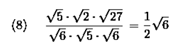# Home | Mathematics | Exponents and Radicals

"Roots" (or "radicals") are the "opposite" operation of applying exponents; we can "undo" a power with a radical, and we can "undo" a radical with a power. For instance, if we square 2, we get 4, and if we "take the square root of 4", we get 2; if we square 3, we get 9, and if we "take the square root of 9", we get 3. In mathematical notation, the previous sentence means the following:

22 = 4, so √4 = 2

32 = 9, so √9 = 3

The "" symbol used above is called the "radical" symbol. The expression " √9 " is read as "root nine", "radical nine", or "the square root of nine".

 Operations:Sum/Subtraction of RadicalsQuotient of Radicals Paper size: US Letter (8.5 x 11 in)   A4 (210 x 297 mm)